#### 期刊菜单

Analysis of a Switching Circuit Based on a Coupled Inductor
DOI: 10.12677/OJCS.2019.83006, PDF, HTML, XML, 下载: 620  浏览: 1,344  科研立项经费支持

Abstract: Combining the coupled inductor with the switching circuit greatly enhances the output voltage gain of the switching circuit and the flexibility of its adjustment. In this paper, the original and secondary sides of the coupled inductor are respectively fused with the diode-capacitor unit. The structure of the switching circuit has been expanded, which provides a certain foundation for the research of new high-performance switching circuits. The detailed analysis and simulation verification of the proposed circuit are carried out. The simulation results verify the correctness of the theoretical analysis.

1. 引言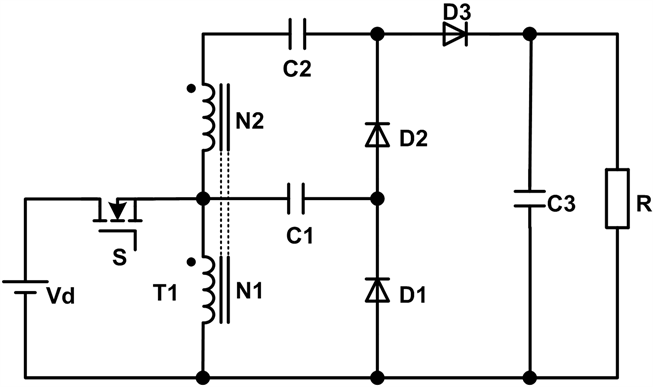Figure 1. The proposed switching circuit

2. 开关电路拓扑结构及其原理分析

CCM模式下，电路主要器件的电流波形如图3所示。具体模态分析如下所述。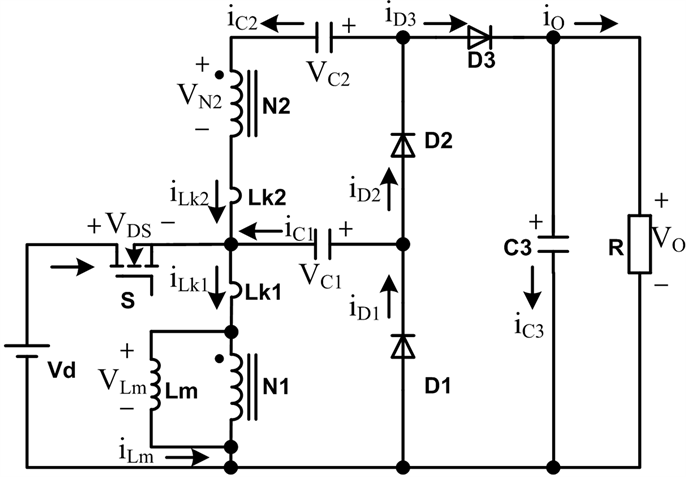Figure 2. Equivalent circuit of switching circuit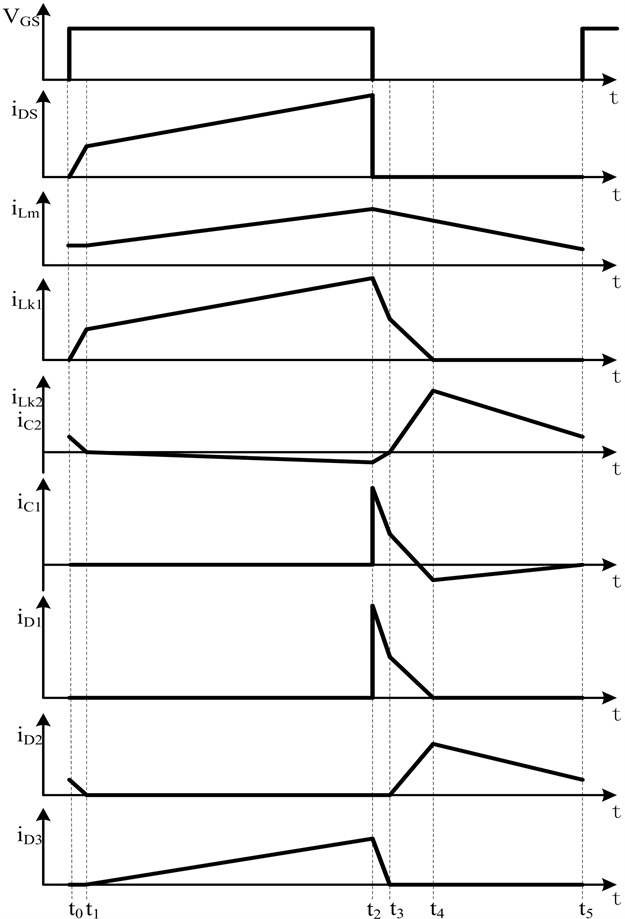Figure 3. The key waveforms in switching circuit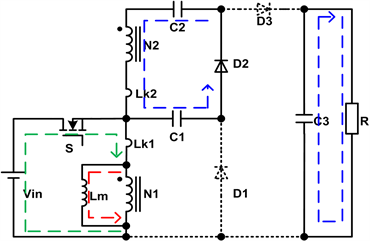(a) 模态1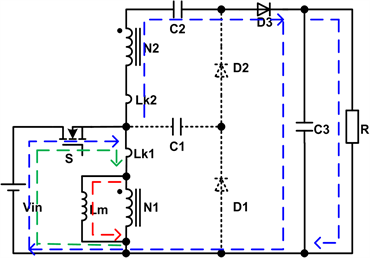(b) 模态2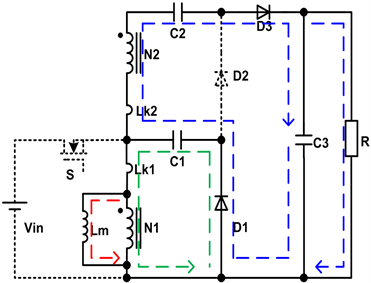(c) 模态3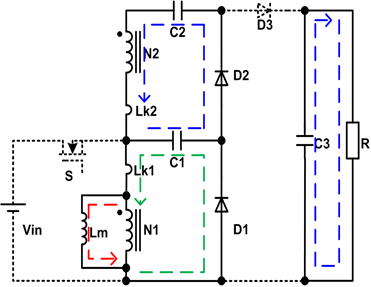(d) 模态4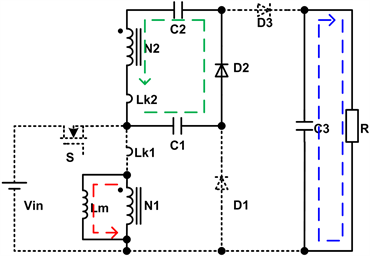(e) 模态5

Figure 4. The modes of circuit in CCM

3. 稳态特性分析

3.1. 变换器电压增益

${v}_{Lm}={V}_{in}$ (1)

${v}_{N2}=n{V}_{in}$ (2)

${v}_{Lm}=-{V}_{C1}$ (3)

${v}_{N2}=-{V}_{C2}+{V}_{C1}$ (4)

${\int }_{0}^{D{T}_{S}}{V}_{in}\text{d}t+{\int }_{D{T}_{S}}^{{T}_{S}}\left(-{V}_{C1}\right)\text{d}t=0$ (5)

${\int }_{0}^{D{T}_{S}}\left(n{V}_{in}\right)\text{d}t+{\int }_{D{T}_{S}}^{{T}_{S}}\left(-{V}_{C2}+{V}_{C1}\right)\text{d}t=0$ (6)

${V}_{C1}=\frac{D}{1-D}{V}_{in}$ (7)

${V}_{C2}=\frac{\left(n+1\right)D}{1-D}{V}_{in}$ (8)

${V}_{o}=\frac{n+1}{1-D}{V}_{in}$ (9)

3.2. 功率器件的电压应力

${V}_{ds}={V}_{in}+{V}_{C1}=\frac{1}{n+1}{V}_{O}$ (10)

${V}_{D1}={V}_{in}+{V}_{C1}=\frac{1}{n+1}{V}_{O}$ (11)

${V}_{D2}={V}_{C2}+{V}_{N2}-{V}_{C1}=\frac{n}{n+1}{V}_{O}$ (12)

${V}_{D3}={V}_{C3}={V}_{o}$ (13)

4. 仿真验证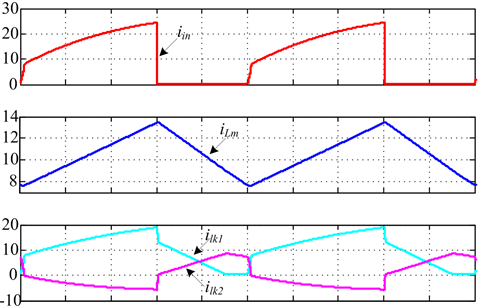(a)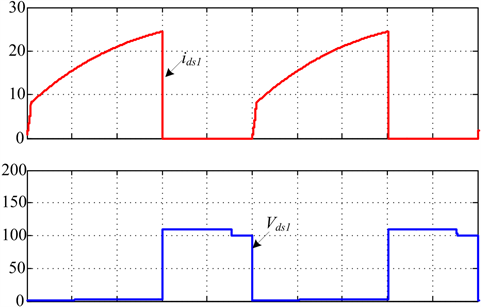(b)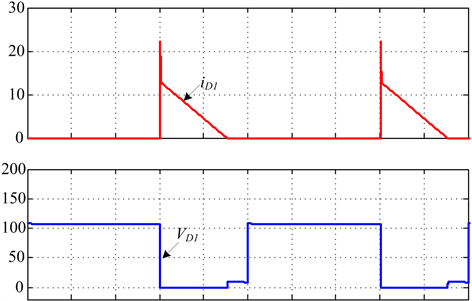(c)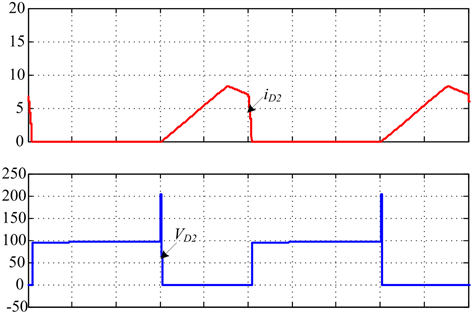(d)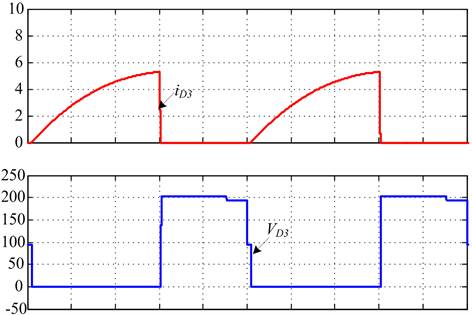(e)

Figure 5. The simulation waveforms of proposed switching circuit

5. 总结

  候世英, 陈剑飞, 孙韬, 张立帅, 邹学伟. 基于Switch-Capacitor网络的单开关升压变换器[J]. 电工技术学报, 2013, 28(10): 206-216.  王琳, 胡雪峰, 代国瑞, 张纯. 一种适于燃料电池发电用高增益Boost变换器[J]. 电源学报, 2014, 12(5): 60-66.  孙良伟, 余运江. 一种适用于光伏发电系统的高增益Boost变换器[J]. 机电工程, 2007, 24(9): 90-93.  Yang, L.-S., Liang, T.-J., Lee, H.C. and Chen, J.F. (2011) Novel High Step-Up DC-DC Converter with Coupled-Inductor and Volt-age-Doubler Circuits. IEEE Transactions on Industrial Electronics, 58, 4196-4206. https://doi.org/10.1109/TIE.2010.2098360  王挺, 汤雨. 应用于微逆变器的高增益DC-DC变换器设计[J]. 电力系统自动化, 2013, 37(13): 121-126.  颜伟鹏, 田书欣, 张永鑫, 等. 小型光伏发电系统中的高增益DC-DC变换器综述[J]. 通信电源技术, 2010, 27(6): 1-5.  王倩倩, 胡雪峰, 李永超, 黄媛媛. 用于可再生能源发电的改进的平方型Boost变换器[J]. 电气应用, 2016, 35(16): 41-46.